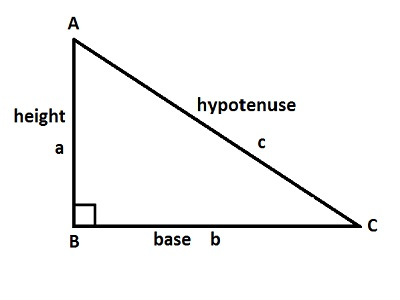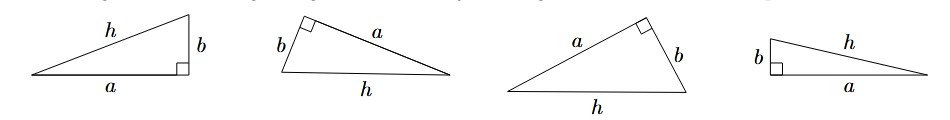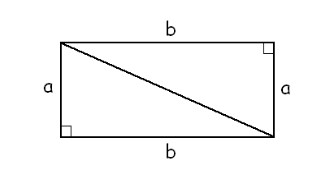# Right Triangle Calculator

Right triangle calculator online tool to calculate area of a right triangle, perimeter of right triangle calculator and hypotenuse of the trianle if the two sides are given.## Pythagorean Theorem

An angle of 90o is called a right angle. Right angles are often denoted with a square arc, as in the examples below. A triangle that has a right angle is called a right triangle. Here are some examples### Perimeter of a Right Triangle

The perimeter is just the length of the boundary. This means that the perimeter of any triangle can be computed by adding the lengths of its three sides.

The perimeter of a triangle ABC can be computed as P = a + b + c ; where a,b and c are the length of the three sides of a trianle.

### Area of Right Triangles

When it comes to area, all we know are rectangles. In other words, every area formula was derived from the area formula of the rectangle.

Every right triangle is half of a rectangle. In other words, given any right triangle, we can use two identical copies of it to form a rectangle. We know how to find the area of the rectangle: A = ab.

Because the rectangle consists of two identical (also called congruent) right triangles, it naturally follows that each takes up half of the area of the rectangle.

Thus the area of the right triangle is A = ab / 2 .### Hypotenuse of a Right Triangles

Note that the side of a right triangle that is opposite the right angle is always longer than the others. This side is called the hypotenuse. The other two sides are called the legs. It is important to identify the hypotenuse of a right triangle - just look at the side opposite the right angle. The pythagorean theorem says that the square of the hypotenuse is equal to the sum of the squares of the legs. In other words,

h2 = a2 + b2 or h = sqrt(a2 + b2)

### Spreading Knowledge Across the World

USA - United States of America  Canada  United Kingdom  Australia  New Zealand  South America  Brazil  Portugal  Netherland  South Africa  Ethiopia  Zambia  Singapore  Malaysia  India  China  UAE - Saudi Arabia  Qatar  Oman  Kuwait  Bahrain  Dubai  Israil  England  Scotland  Norway  Ireland  Denmark  France  Spain  Poland  and  many more....